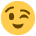# High Low Method

Am really struggling with this question, not sure where to start,

Any help would be appreciated

Thanks Emma

Use the high low method to predict the costs at a production level of 8,000 units
Production level

Units Total cost
£
5,000 15,500
7,000 19,500
9,000 23,500
11,000 27,500

Units Cost £

£ Variable unit cost

£ Fixed cost

£ Production cost for 8,000 units

• High 11000 units £27500
Low 5000 units £15500
= 6000 units £12000

£12000/6000 units= £2 variable unit cost
£2 x 5000 units= £10000 variable cost

£15500 total cost
-£10000 variable cost
=£5500 fixed cost

8000 units x £2 =£16000 variable cost
+£5500 fixed cost
=£21500 total cost
• I don't follow the exact higher and lower method, I use any two. Here take the first two production level cost and use the difference value between them. Below is illustration:
U= Units
£= value

Step 1-
7000U-5000U= 2000U
£19500-£15500=£4000
i.e. 2000U =£4000
Or 1U= £2 ( this is the variable cost per unit)

Step 2-
You should know total variable + total fixed= total cost.
Or total fixed = total cost- total variable

Now use this variable unit cost and formula to find a fixed costs by taking Any of the production level figure. All should result in same answer.
Let's use 5000U=£15500
Fixed cost = £15500-( 5000×£2)= £15500-£10000 = £5500
So, £5500 is a fixed cost and will be charged to any production level.

Step 3-
Now at last, let's find the cost of 8000U.
Fixed cost= £5500
Variable cost= £2×8000=£16000
Total cost= £21500.